# How To Use Seaborn Color Palette to Color Boxplot?

• Difficulty Level : Easy
• Last Updated : 12 Nov, 2020

Box Plot is the visual representation of the depicting groups of numerical data through their quartiles. Boxplot is also used to detect the outlier in the data set. It captures the summary of the data efficiently with a simple box and whiskers and allows us to compare easily across groups.

Adding the right set of colors to Boxplot can reveal a lot of different patterns that were not seen before.  Seaborn Color Palette makes it really easy to add colors in Boxplot. This article will explain how to use the Seaborn color palette to color Boxplots.

There are 2 ways of coloring Boxplot using the Seaborn color palette

### 1) Using predefined palettes of seaborn

This can be done by adding a palette argument inside the boxplot() function and giving it any predefined seaborn color palette value like “Set1”, “Set2”, “Paired”, “Set3” etc.

Step 1: Creating a Dataframe.

## Python3

 `# import the required library``import` `seaborn as sns ``import` `matplotlib.pyplot as plt``import` `pandas as pd``import` `numpy as np`` ` `#Generate some random data``np.random.seed(``45``)``df ``=` `pd.DataFrame({``    ``'Corn'``: np.random.normal(``40``, ``15``, ``100``),``    ``'Rice'``: np.random.normal(``60``, ``10``,``100``),``    ``'Wheat'``: np.random.normal(``80``, ``5``, ``100``),``    ``'Peas'``: np.random.normal(``30``, ``13``, ``100``),``})``print``(df)`

Output: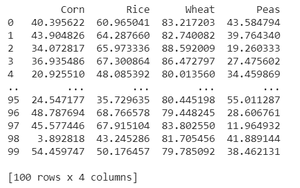Step 2: Use pandas.melt() to convert wide to long

## Python3

 `# Since the above data is in wide``# form we convert it into long``# form using melt function``data_df ``=` `df.melt(var_name``=``'Pulses'``,``                  ``value_name``=``'Tons Consumed'``)``print``(data_df)`

Output: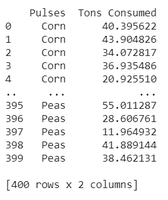Step 3: Create a boxplot to use a palette.

## Python3

 `# Create boxplot and add palette``# with predefined values like Paired, Set1, etc``sns.boxplot(x``=``"Pulses"``, y``=``"Tons Consumed"``,``            ``data``=``data_df, palette``=``"Paired"``)`

Output: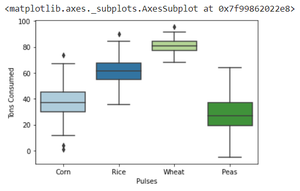Using different color:

## Python3

 `sns.boxplot(x``=``"Pulses"``, y``=``"Tons Consumed"``,``            ``data``=``data_df, palette``=``"Set1"``)`

Output: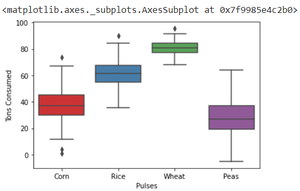Possible palette values are:

Accent, Accent_r, Blues, Blues_r, BrBG, BrBG_r, BuGn, BuGn_r, BuPu, BuPu_r,

CMRmap, CMRmap_r, Dark2, Dark2_r, GnBu, GnBu_r, Greens, Greens_r, Greys, Greys_r,

OrRd, OrRd_r, Oranges, Oranges_r, PRGn, PRGn_r, Paired, Paired_r, Pastel1, Pastel1_r,

Pastel2, Pastel2_r, PiYG, PiYG_r, PuBu, PuBuGn, PuBuGn_r, PuBu_r, PuOr, PuOr_r, PuRd,

PuRd_r, Purples, Purples_r, RdBu, RdBu_r, RdGy, RdGy_r, RdPu, RdPu_r, RdYlBu, RdYlBu_r,

RdYlGn, RdYlGn_r, Reds, Reds_r, Set1, Set1_r, Set2, Set2_r, Set3, Set3_r, Spectral,

Spectral_r, Wistia, Wistia_r, YlGn, YlGnBu, YlGnBu_r, YlGn_r, YlOrBr, YlOrBr_r, YlOrRd,

YlOrRd_r, afmhot, afmhot_r, autumn, autumn_r, binary, binary_r, bone, bone_r, brg, brg_r,

bwr, bwr_r, cividis, cividis_r, cool, cool_r, coolwarm, coolwarm_r, copper, copper_r, cubehelix

### 2) Manually creating your own color palette and using it

Approach:

• Create your own array of colors.
• Use the set_palette() function of seaborn and add your array name as an argument.
• Call the boxplot() function to make the boxplot
•

## Python3

 `#create your own color array``my_colors ``=` `[``"#9b59b6"``, ``"#3498db"``, ``             ``"#2ecc71"``, ``"#006a4e"``]`` ` `# add color array to set_palette``# function of seaborn``sns.set_palette( my_colors )`` ` `# make boxplot``sns.boxplot( x ``=` `"Pulses"``, y ``=` `"Tons Consumed"``,``            ``data ``=` `data_df)`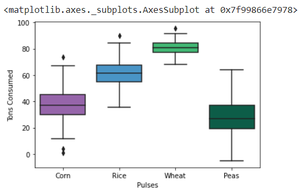My Personal Notes arrow_drop_up